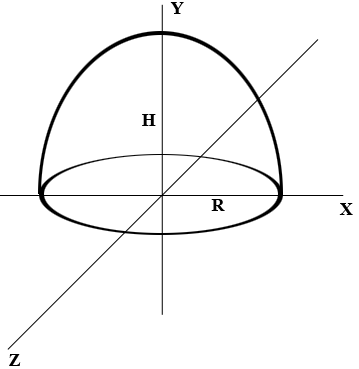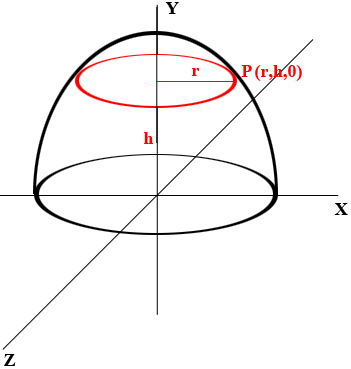SEARCH HOMEMath Central Quandaries & QueriesQuestion from Joel: Find a formula to calculate the circumferences of an inverted bowl at various heights. E.g. Take an inverted bowl with a diameter of "x" cm and a depth / height of "y" cm. How can I calculate the circumferences at distances of various heights above the base? Alternately, what would be the formula to calculate the distance of the line segment resulting from a line which intersects both sides of a parabola in which that line is drawn parallel to the tangent of the vertex of the parabola at any given distance from the vertex?Hi Joel,

Here is my diagram of your inverted bowl. The cross section in the XY-plane is a parabola opening downwards so its equation is

$y = a x^2 + b$

where $a$ and $b$ are real numbers.I have made the height of the bowl $H$ cm and the radius of the case $R$ cm. Hence in the XY-plane $(0, H)$ and $(R, 0)$ are on the parabola and hence $b = H$ and $a = - \large \frac{H}{R^2}$ and thus the equation of the parabola is

$y = - \frac{H}{R^2} x^2 + H.$

Now suppose you slice the bowl by a plane which is $h$ cm above the base to obtain a circle of radius $r$ cm.The point $(r, h)$ in the XY-plane is on the parabola and hence

$h= - \frac{H}{R^2} r^2 + H.$

Solving for $r$ yields

$r = \sqrt{\frac{H-h}{H}} R.$

Using $r$ you can calculate the circumference of the red circle in the diagram above.

PennyMath Central is supported by the University of Regina and The Pacific Institute for the Mathematical Sciences.